# Shading Fractions, Decimals and Percentages (2)

In this worksheet, students identify the fraction, decimal or percentage shaded in a rectangle.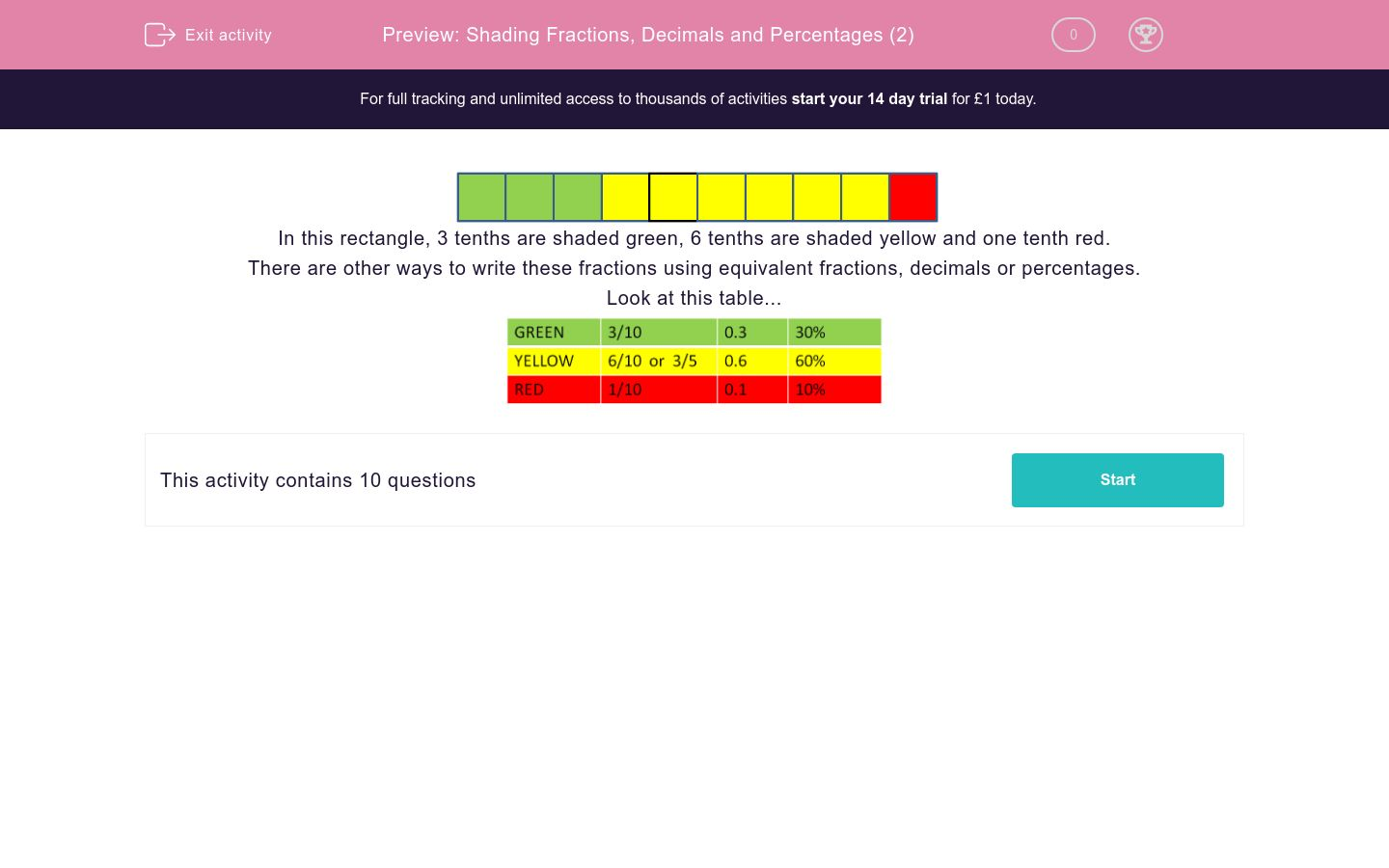Key stage:  KS 2

Curriculum topic:   Maths and Numerical Reasoning

Curriculum subtopic:   Decimals

Difficulty level:### QUESTION 1 of 10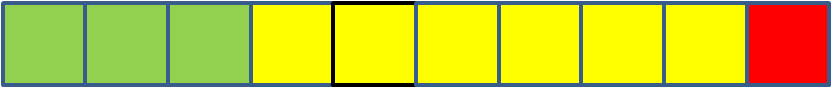In this rectangle, 3 tenths are shaded green, 6 tenths are shaded yellow and one tenth red.

There are other ways to write these fractions using equivalent fractions, decimals or percentages.

Look at this table...Look at the shading in this rectangle.What decimal fraction is shaded yellow?

0.6

0.3

6

Look at the shading in this rectangle.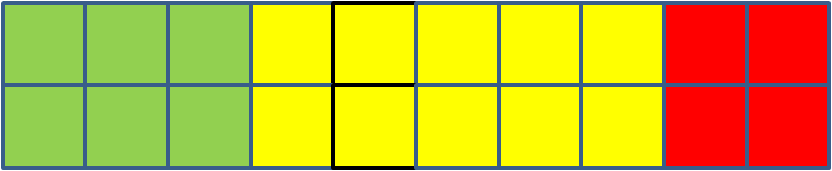20

2

4

Look at the shading in this rectangle.1/2

3 tenths

3/5

Look at the shading in this rectangle.1/2

10 tenths

3/5

Look at the shading in this rectangle.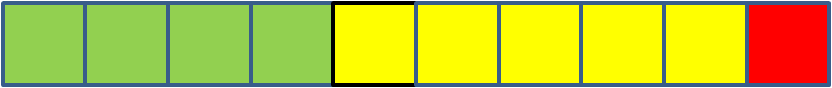1/2

4/5

2/5

Look at the shading in this rectangle.30

6

60

Look at the shading in this rectangle.What decimal fraction is shaded green?

0.3

0.6

6

Look at the shading in this rectangle.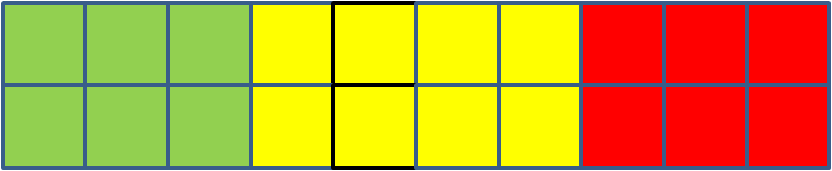What decimal fraction is shaded red?

0.6

0.3

6

Look at the shading in this rectangle.1/2

0.8

2/5

Look at the shading in this rectangle.8/10

40

80

• Question 1

Look at the shading in this rectangle.What decimal fraction is shaded yellow?

0.6
• Question 2

Look at the shading in this rectangle.20
• Question 3

Look at the shading in this rectangle.1/2
• Question 4

Look at the shading in this rectangle.1/2
• Question 5

Look at the shading in this rectangle.2/5
• Question 6

Look at the shading in this rectangle.30
• Question 7

Look at the shading in this rectangle.What decimal fraction is shaded green?

0.3
• Question 8

Look at the shading in this rectangle.What decimal fraction is shaded red?

0.3
• Question 9

Look at the shading in this rectangle.2/5
• Question 10

Look at the shading in this rectangle.40
---- OR ----

Sign up for a £1 trial so you can track and measure your child's progress on this activity.

### What is EdPlace?

We're your National Curriculum aligned online education content provider helping each child succeed in English, maths and science from year 1 to GCSE. With an EdPlace account you’ll be able to track and measure progress, helping each child achieve their best. We build confidence and attainment by personalising each child’s learning at a level that suits them.

Get started